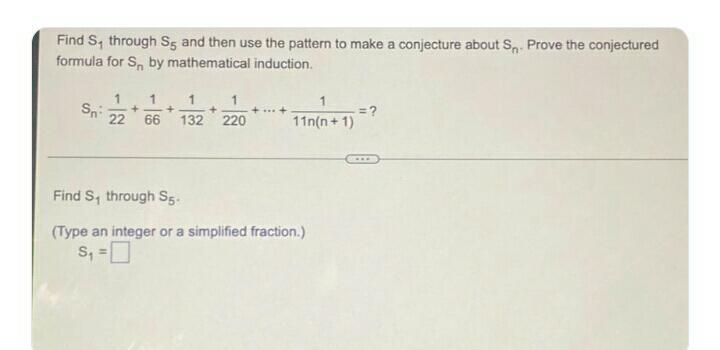Question:

# Find S, through Sg and then use the pattern to make a conjecture about S, Prove the conjectured formula for S, by mathematical iFind S, through Sg and then use the pattern to make a conjecture about S, Prove the conjectured formula for S, by mathematical induction Sn: 1 1 1 1 + + +++ = ? 22 66 132 220 11n(n+1) + Find S, through S5 (Type an integer or a simplified fraction.) S =